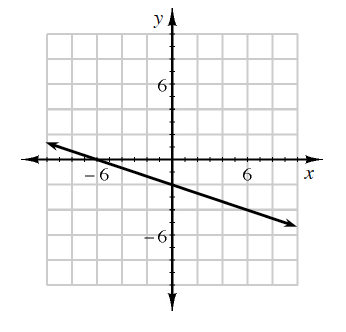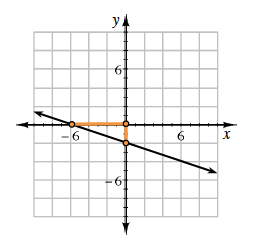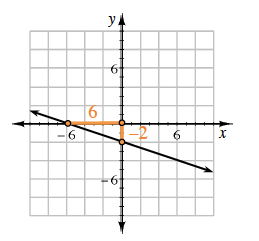### Home > CCA > Chapter 3 > Lesson 3.1.2 > Problem3-22

3-22.

For the line graphed at right:1. Determine the slope.

Start by selecting two points on the line, such as $(6,0)$ and $(0,−2)$.
Use them to find the rise and the run and then calculate the slope.

On the line graph a triangle is drawn with the horizontal leg from (negative 6, comma 0) to (0, comma 0) and the vertical leg from (0, comma 0) to (0, comma negative 2).

Divide the rise, $−2$, by the run, $6$, to determine the slope.

On the triangle the horizontal leg is labeled 6 and the vertical leg is labeled negative 2.

$-\frac{2}{6}\text{ or }-\frac{1}{3}$

2. Find the equation of the line.

Use the $y$-intercept for $b$ and the slope for $m$ in the equation $y=mx+b$.Use the eTool below to help solve the problem.
Click the link at right for the full version of the eTool: CCA 3-22 HW eTool